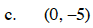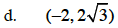### Home > CALC > Chapter 9 > Lesson 9.4.1 > Problem9-115

9-115.
1. For each of the rectangular coordinates below, find two equivalent polar coordinates. Homework Help ✎

1. (3, −3)

2. (0, 0)

3. (0, −5)

4.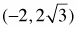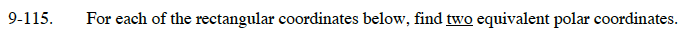Graph each point and draw a segment connecting the point to the origin. Create a right triangle to determine the length and direction of the segment.

Recall that each point can be written four ways: (+, +), (+, –), (–, –), and (–, +)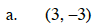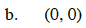It the radius is 0, does it matter what the angle is?